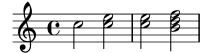### 3.2.1 Music expressions explained

In LilyPond input files, music is represented by music expressions. A single note is a music expression:

```a'4
```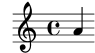Enclosing music in braces creates a compound music expression. Here we have created a compound music expression with two notes:

```\relative { a'4 g4 }
```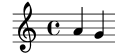Putting a group of music expressions (e.g., notes) in braces means that they are in sequence (i.e., each one follows the previous one). The result is another music expression:

```\relative { { a'4 g } f4 g }
```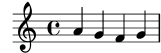#### Analogy: mathematical expressions

This mechanism is similar to mathematical formulas: a big formula is created by composing small formulas. Such formulas are called expressions, and they can contain other expressions, so you can make arbitrarily complex and large expressions. For example,

 ```1 1 + 2 (1 + 2) * 3 ((1 + 2) * 3) / (4 * 5) ```

This is a sequence of expressions, where each expression is contained in the next (larger) one. The simplest expressions are numbers, and larger ones are made by combining expressions with operators (like +, *, and /) and parentheses. Like mathematical expressions, music expressions can be nested arbitrarily deep, which is necessary for complex music like polyphonic scores.

#### Simultaneous music expressions: multiple staves

Music Glossary: polyphony.

This technique is useful for polyphonic music. To enter music with more voices or more staves, we combine expressions in parallel. To indicate that two voices should play at the same time, simply enter a simultaneous combination of music expressions. A ‘simultaneous’ music expression is formed by enclosing expressions inside `<<` and `>>`. In the following example, three sequences (all containing two separate notes) are combined simultaneously:

```<<
\relative { a'2 g }
\relative { f'2 e }
\relative { d'2 b }
>>
```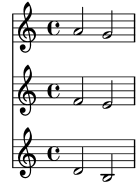Note that we have indented each level of the input with a different amount of space. LilyPond does not care how much (or little) space there is at the beginning of a line, but indenting LilyPond code like this makes it much easier for humans to read.

Note: Each note is relative to the previous note in the input; the first one in a `\relative` block is relative to ‘c’ (one octave below the middle C).

#### Simultaneous music expressions: single staff

To determine the number of staves in a piece, LilyPond looks at the beginning of the first expression. If there is a single note, there is one staff; if there is a simultaneous expression, there is more than one staff. The following example shows a complex expression, but as it begins with a single note it will be set out on a single staff.

```\relative {
c''2 <<c e>> |
<< { e2 f } { c2 <<b d>> } >> |
}
```